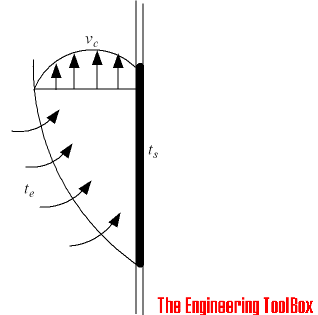Engineering ToolBox - Resources, Tools and Basic Information for Engineering and Design of Technical Applications!

# Convective Heat Transmission - Air Velocity and Air Flow Volume

## Hot or cold vertical surfaces generates vertical air flows - calculate air velocity and volume flow.

A hot or cold vertical surface will generate a vertical upwards or downwards air flow as indicated in the figure below.The air velocity in the center of a convective air flow in parallel to the hot or cold surface - at a vertical distance - can be estimated as:

### Air Velocity

vc = 0.65 [g l dt / (273 + te)]1/2                                              (1)

where

vc = velocity in center of airflow (m/s)

g = acceleration of gravity (9.81 m/s2)

l = vertical distance from bottom (or top) of the surface (m)

dt = te - ts= temperature difference between surface and room environment

ts = surface temperature (oC)

te = surrounding environment - ambient - temperature (oC)

For a cold surface where the air will flow downwards - the value will be negative.

### Air Volume

Convective air flow volume at a vertical distance generated by the hot or cold surface can be calculated as

Q = 0.019 [g (te - ts) / (273 + te)]0.4 l1.2                                            (2)

where

Q = air flow volume (m3/s per meter surface width)

### Example - Convective Air Flow from a Cold Window

The air velocity at the lower level of a cold (5oC) window with height 2 m in a room with temperature 20oC can be calculated as

vc = 0.65 [(9.81 m/s2) (2 m) ((20 oC) - (5 oC)) / (273 + (20 oC))]1/2

= 0.65 m/s

The air flow volume (for a window with width 1m) can be calculated as

Q = 0.019 [(9.81 m/s2) ((20 oC) - (5 oC) / (273 + (20 oC))]0.4 (2 m)1.2

= 0.033 m3/s

= 119 m3/h

### Example - A Heating Element (or Radiator) and Convective Air Flow

The air flow velocity at the top of a hot (80oC) radiator with height 1 m in a room with temperature 20oC can be calculated as

vc = 0.65 [(9.81 m/s2) (1 m) ((80 oC) - (20 oC) / ((273 K) + (20 oC))]1/2

= 0.92 m/s

The air flow volume (for radiator with width 1 m) can be calculated as

Q = 0.019 [(9.81 m/s2) ((80 oC) - (20 oC) / ((273 K) + (20 oC))]0.4 (1 m)1.2

= 0.025 m3/s

= 90.4 m3/h

## Related Topics

• ### Heating

Heating systems - capacity and design of boilers, pipelines, heat exchangers, expansion systems and more.

## Related Documents

• ### Convective Air Flow - Single Heat Source

Calculate the vertical air flow and air velocity generated by a single heat source.
• ### Convective Air Flows from Typical Heat Sources

Convective air flows from typical heat sources like people, computers, radiators and more.
• ### Convective Heat Transfer

Heat transfer between a solid and a moving fluid is called convection. This is a short tutorial about convective heat transfer.
• ### Steam Radiators and Convectors - Heating Capacities

Steam radiators and steam convectors - heating capacities and temperature coefficients.

## Search

Search is the most efficient way to navigate the Engineering ToolBox.

## Engineering ToolBox - SketchUp Extension - Online 3D modeling!

Add standard and customized parametric components - like flange beams, lumbers, piping, stairs and more - to your Sketchup model with the Engineering ToolBox - SketchUp Extension - enabled for use with older versions of the amazing SketchUp Make and the newer "up to date" SketchUp Pro . Add the Engineering ToolBox extension to your SketchUp Make/Pro from the Extension Warehouse !

We don't collect information from our users. More about

## Citation

• The Engineering ToolBox (2005). Convective Heat Transmission - Air Velocity and Air Flow Volume. [online] Available at: https://www.engineeringtoolbox.com/convective-air-flow-d_1006.html [Accessed Day Month Year].

Modify the access date according your visit.

11.23.12